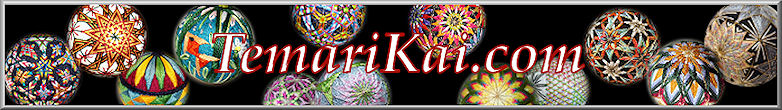#Mari Sizes - Diameter vs Circumference; Inches vs Centimeters

The international reach of Temari creates some confusion when it comes to specifying mari size. North America by culture and custom uses Imperial measurement (inches, feet, yards), and by custom describes the size of "round things" using diameter, which is the distance through the object at its widest point. The rest of the world, uses Metric measurement (millimeters, centimeters, meters) and usually defines the size of "round things" by circumference, which is the distance around the perimeter of the circle. More than a few times people on both sides of the world have been caught trying to make a diameter measurement as circumference, and vice versa; (not an easy thing to pull off if you think about it). By convention, given that the Japanese books are metric-based, we use circumference in cm for measuring temari. Additionally, this solves the problem of "how do I measure the diameter of a ball when I can't stick a ruler through it?" (yes, that question has been asked, more than once....) - it is much easier to measure the circumference (just wrap the tape measure around the widest of the ball)

The best choice for temari crafting is metric, for several reasons: it is the system used in the Japanese books, and metric measurements are much easier to use (honest). Remember, the preferred manner to describe distance in temari patterns is relative, rather than absolute. This means describing a distance as say, "1/2 of the distance from pole to equator", or some other relative value of two landmarks on the mari, rather than say "1/2 inch" or "3cm". Therefore, the use of actual absolute measurement usually is limited to specifying mari sizes and spacing out 10 Combination divisions. No matter what you are used to, using millimeters (mm) and centimeters (cm) is so much easier, since one can divide and convert very simply. Virtually all rulers and tape measures are printed with dual units, both Imperial (also known as "English") and metric; the V-Rulers and C10 gauges used for 10 Combination dividing are marked in mm. Since almost every ruler and tape measure  has both inch and cm/mm markings, it requires nothing other than taking on the switch, and it makes things so very much easier.

When the occasion might arise that a conversion needs to be done to "translate" a diameter measurement in inches to circumference in cm, we only need to remember from school that the circumference (the distance around the ball at its widest) is obtained by measuring the diameter (the distance through the center of the ball at its widest) and multiplying by "Pi" - a mathematical constant of 3.14 (you don't need to understand why, just know that it works).  The other needed info is the conversion between inches and centimeters (1 inch = 2.54 cm)  While it may seem straightforward to figure out diameter in inches from circumference in centimeters (and vice versa)  -  most people would rather chew nails. To help out, here is a quick reference to convert common mari sizes between diameter in inches to circumference in centimeters. They are figured to the nearest half-inch; you'll notice that for every half-inch in diameter, the circumference increases by about 4cm. It's straightforward and common sense to determine a quarter-inch up or down (add or subtract 2 cm from the closest). Likewise if you need to convert something larger, just add accordingly by 4cm per inch.

 Conversion between Diameter (inches) and Circumference (cm) for Mari Sizing Diameter in Inches Circum. in CM 2 16 2.5 20 3 24 3.5 28 4 32 4.5 36 5 40 5.5 44 6 48 6.5 52 7 56 7.5 60 8 64